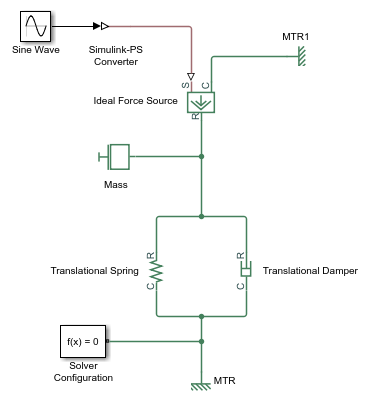# simscape.logging.Node

Hierarchy tree for simulation data

## Description

`simscape.logging.Node` represents the hierarchy of nodes for logging simulation data in a model. The tree starts with the workspace variable, which represents simulation data for the whole model, and recursively creates nodes for each of the children.

The children depend on the type of the parent node:

• For the top-level simulation log workspace variable, the children are all the Simscape™ blocks (and subsystems containing Simscape blocks) in the top-level model diagram.

• For a subsystem or a structural block, the children are all the constituent Simscape blocks and subsystems.

• For a block, the children are all its physical ports, Through and Across variables, and all internal variables defined in the block's Simscape file.

• For a physical port, the children are all its Across variables.

Final nodes in this recursion correspond to all the variables logged for the model. Final nodes do not have children nodes, and contain the series data logged during simulation.

## Creation

This object is created automatically during simulation, as part of the simulation log workspace variable, if you enable data logging for the model.

## Properties

expand all

The name identifying the `Node` object. For the simulation log workspace variable, this is the name of the top-level block diagram. For blocks and subsystems, the `id` is constructed automatically as a valid MATLAB® identifier based on the name of the block or subsystem. For other types, the `id` is the name of the corresponding port or variable.

Logical value that indicates how you can reuse logged simulation data in a future session. If `savable` is `1`, use the regular MATLAB interface to save the workspace variable as a MAT-file and load a MAT-file into a variable. This property depends on the logging method of the `Node` object. For more information, see Saving and Retrieving Logged Simulation Data.

Logical value that indicates how you can reuse logged simulation data in a future session. If `exportable` is `1`, use `simscape.logging.export` and `simscape.logging.import`. This property depends on the logging method of the `Node` object. For more information, see Saving and Retrieving Logged Simulation Data.

For `Node` objects that do not have children nodes, and therefore correspond to the logged variables, the `series` property returns a `simscape.logging.Series` object that contains the simulation series data for this variable. For nodes that do not represent variables, the `series` property is hidden. If you access the hidden `series` property for such node, the property returns a `simscape.logging.Series` object representing an empty series (with zero points).

The other properties are dynamic, and represent all the children of the `Node` object.

## Object Functions

 `get` Access node of simulation logging data tree using slash-delimited path `getSource` Navigate from node object to block that generated it `plot` Plot all series associated with Node object `plotxy` Plot series associated with two node objects against each other `print` Print complete logging tree of Node object

## Examples

collapse all

Plot complete logging tree for a model, and then plot velocities of all the blocks in the model.

Consider the following model. The model name is `simple_mech2`, and data logging is enabled with the default workspace variable name, `simlog`.Print the complete logging tree for the model:

```print(simlog) ```
``` simple_mech2 +-Ideal_Force_Source | +-C | | +-v | +-R | | +-v | +-S | +-f | +-v +-MTR | +-V | | +-v | +-f +-MTR1 | +-V | | +-v | +-f +-Mass | +-M | | +-v | +-f +-Simulink_PS_Converter +-Translational_Damper | +-C | | +-v | +-R | | +-v | +-f | +-v +-Translational_Spring +-C | +-v +-R | +-v +-f +-v +-x```

Plot velocities of all the blocks in the model:

```plot(simlog,'units','m/s','depth',2) ```

This command filters simulation data in two ways. It plots only series that are commensurate with units `m/s` (that is, velocities), based on the `units` argument. And because of the `depth` argument, it plots only those velocity variables that are associated with the block itself. If you refer to the logging tree, only the Ideal Force Source, Translational Damper, and Translational Spring blocks have a velocity (`v`) variable at the second level. Because of the `depth` argument, velocities of the block ports (one level down) do not get plotted.

## Version History

Introduced in R2010b